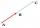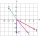# Line

Line p passing through A[-10, 6] and has direction vector v=(3, 2). Is point B[7, 30] on the line p?

Result

#### Solution:Leave us a comment of example and its solution (i.e. if it is still somewhat unclear...):Be the first to comment!#### To solve this verbal math problem are needed these knowledge from mathematics:

For Basic calculations in analytic geometry is helpful line slope calculator. From coordinates of two points in the plane it calculate slope, normal and parametric line equation(s), slope, directional angle, direction vector, the length of segment, intersections the coordinate axes etc. Two vectors given by its magnitudes and by included angle can be added by our vector sum calculator.

## Next similar examples:

1. Three points 2The three points A(3, 8), B(6, 2) and C(10, 2). The point D is such that the line DA is perpendicular to AB and DC is parallel to AB. Calculate the coordinates of D.
2. Find the 5Find the equation with center at (1,20) which touches the line 8x+5y-19=0
3. Find the 10Find the value of t if 2tx+5y-6=0 and 5x-4y+8=0 are perpendicular, parallel, what angle does each of the lines make with the x-axis, find the angle between the lines?
4. Linear independenceDetermine if vectors u=(-4; -5) and v=(20; 25) are linear Linear dependent.
5. CuboidsTwo separate cuboids with different orientation in space. Determine the angle between them, knowing the direction cosine matrix for each separate cuboid. u1=(0.62955056, 0.094432584, 0.77119944) u2=(0.14484653, 0.9208101, 0.36211633)
6. Two forcesTwo forces with magnitudes of 25 and 30 pounds act on an object at angles of 10° and 100° respectively. Find the direction and magnitude of the resultant force. Round to two decimal places in all intermediate steps and in your final answer.An airplane leaves an airport and flies due west 120 miles and then 150 miles in the direction S 44.1°W. How far is the plane from the airport (round to the nearest mile)?The rectangular crossroads comes passenger car and an ambulance, the ambulance left. Passenger car is at 43 km/h and ambulance 52 km/h. Calculate such a relative speed of the ambulance moves to the car.
9. Bearing - navigationA ship travels 84 km on a bearing of 17°, and then travels on a bearing of 107° for 135 km. Find the distance of the end of the trip from the starting point, to the nearest kilometer.
10. TriangleTriangle KLM is given by plane coordinates of vertices: K[14, -2] L[8, 13] M[-1, -18]. Calculate its area and itsinterior angles.
11. Angle between vectorsFind the angle between the given vectors to the nearest tenth of a degree. u = (-22, 11) and v = (16, 20)
12. Unit vector 2DDetermine coordinates of unit vector to vector AB if A[-6; 8], B[-18; 10].
13. Vector sumThe magnitude of the vector u is 12 and the magnitude of the vector v is 8. Angle between vectors is 61°. What is the magnitude of the vector u + v?
14. 3d vector componentThe vector u = (3.9, u3) and the length of the vector u is 12. What is is u3?
15. Scalar productCalculate the scalar product of two vectors: (2.5) (-1, -4)
16. VectorCalculate length of the vector v⃗ = (9.75, 6.75, -6.5, -3.75, 2).
17. VectorsVector a has coordinates (8; 10) and vector b has coordinates (0; 17). If the vector c = b - a, what is the magnitude of the vector c?